10.8 Physics experiment: simulation of moving electrons, hydrogen and boron ions in a magnetic field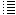Computer simulation of idea 10

Experiment 8

The problem: We want an amount of electrons circulating in a nice circle inside the vacuum chamber. How do we get them there?

Fig.1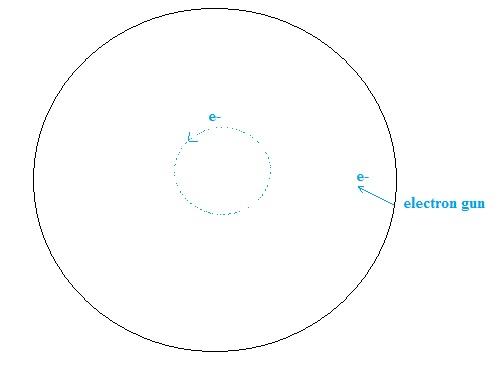Fig.2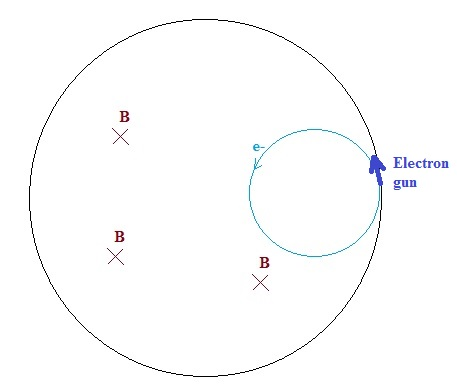If we have an uniform magnetic field perpendical to the plane in which the electrons are moving and we inject the electrons in a point near the wall,
then they will circulate in a circle near the wall of the vacuum chamber, see fig. 2. We do not want this.

Fig. 3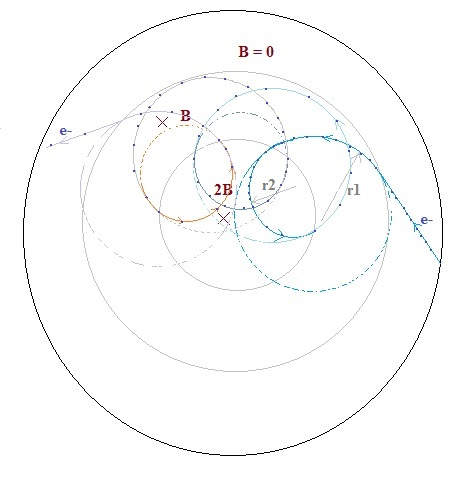Let's now make three regions inside the vacuum chamber with different B-fields: in the centre area 2B, then a circular region with B and then a circular region with zero B-field.
The electrons are injected in a point near the wall and first travel in a straight line because there is no magnetic field. Then they enter in the region with field B and their trajectory
will be curved (radius r1= mv/Bq).  After a while they will enter into the region with field 2B and their trajectory will be even more curved (r2=0.5.r1). In fig. 3 their trajectory
through the vacuum chamber is drawn (the radii are arbitrarily choosen, although r2=0.5*r1,  and are not in scale). Here the electrons finally leave the chamber.

Mathematically an electron would only stay in a circular region with a determined magnetic field, if it enters this region via the tangent, but then it will not enter...

Below tried again with three regions with different B-fields.

Fig. 4a & Fig. 4b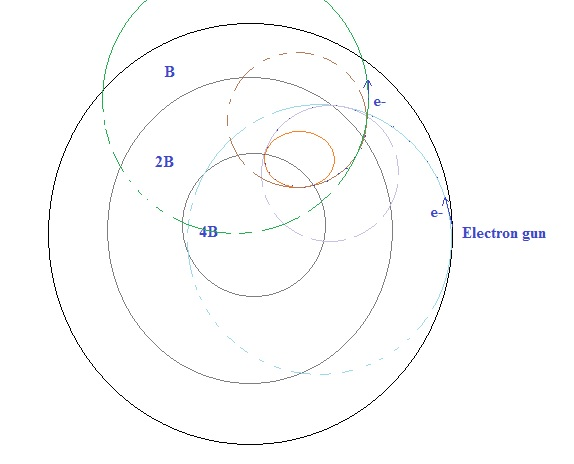What happens if the magnetic field in the centre is less than farther away from the centre?

Fig. 5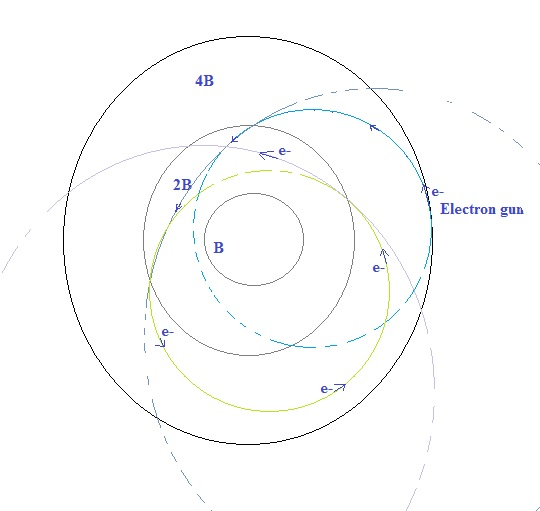Again they fly away...

I have tried in the simulation program with various angles of the electron gun, and various magnetic field configurations (for example from the centre
outwards first linear increasing till some distance from the centre, and then linear decreasing until zero, etc.), but I could not manage to trap the electrons
in an orbit near the centre.

And if we decrease the speed of the electrons with an electric field?

Fig. 6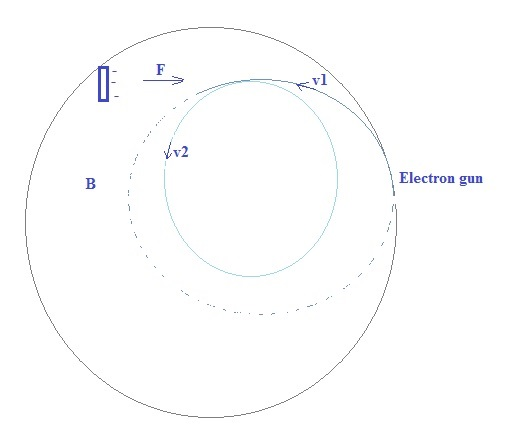This is perhaps a possibility...

 (Jan. 2013) (Jan. 2013) (Jan. 2013) (3 Febr. 2013) (6 Febr. 2013) (8 Febr. 2013) (16 Jan. 2014) (17 Jan. 2014) (20 Jan. 2014) ( Febr. 2014- 2016) Computer simulation tenth idea (2014-2016) Eleventh idea   (2016.. 2017)2016      by  Rinze Joustra        www.valgetal.com